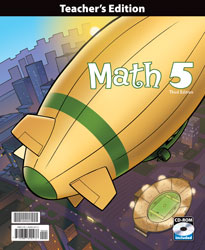## BJU Math 5 Teacher's Edition with CD (3rd ed.)

• ₱2,160.00
• Save ₱2,090

Math 5 (3rd ed.) uses manipulatives to build on the mathematical foundations of multiplication, division, and place value, and to develop the student’s understanding of geometry, fractions, decimals, ratios, and measurements. Students read and interpret a variety of graphs and charts. The practical use of the Problem-Solving Plan is emphasized as well as mental math computation and the memorization of addition, subtraction, multiplication, and division facts.

• Edition - 3rd ed.
• Item Code - 269522

We Also Recommend